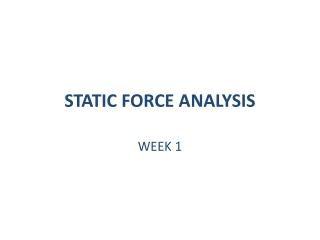DownloadDownload PresentationSTATIC FORCE ANALYSIS

# STATIC FORCE ANALYSIS

Télécharger la présentation## STATIC FORCE ANALYSIS

- - - - - - - - - - - - - - - - - - - - - - - - - - - E N D - - - - - - - - - - - - - - - - - - - - - - - - - - -
##### Presentation Transcript

1. STATIC FORCE ANALYSIS WEEK 1

2. COUPLE MOMENT OF COUPLE Couple: Two equal and opposite forces (F & F’) Moment of Couple: A vector normal to the plane of the couple (M=RBAX F)

3. Conditions of Equilibrium A system of bodies is in equilibrium, if, and only if: In 2-D (planar) systems:

4. Two-Force Members Not in equilibrium F0, M0 Not in equilibrium F=0, M0 In equilibrium F=0, M=0 Condition of equilibrium, for any two-force member with no applied torque: The forces are equal, opposite and have the same line of action.

5. Three-Force Members O Not in equilibrium M0 In equilibrium F=0, M=0 Condition of equilibrium, for any three-force member with no applied torque: Forces should be coplanar Four-Force Members:The problem is reduced to one of three-force member. Then the approach above is applied.

6. Example (Graphical Solution) a) Link 3 is a two-force member C

7. 1 N=0.225 lb b) Link 4 is a three-force member c )1 st approach: OR c’ ) 2 nd approach: Since link 4 is is a three-force member; lines of action of forces P, F34 should intersect at a point . Therefore direction of F14 F14 is obtained. d) Force triangle is used. If 1st approach was used, this triangle gives direction and magnitude of If 2nd approach was used, this triangle gives magnitudes of F34, F14 Since, graphically F14 e) Link 3// Action and reaction forces are equal: and f)Link 2// F=0

8. (Analytical Solution) 4// = 5(cos68.4i+sin68.4j) X 120(-cos40i-sin40j) +12(cos68.4i+sin68.4j) X F34(cos 22.4i+sin 22.4j) = 0 F34 = 33.1 lb 2//

9. Problem (AnalyticalSolution) (withoutfriction) O2A=75 mm P=0.9 kN AB=350 mm M12=? 2 3 4 4// F=0 F34+P+F14=0 F34(cos11,95i-sin11,95j)-900i+F14j=0 i: 0.978 F34 = F34x= 900 F34 = 920.25 N j: 0.207 F34 = F34y= F14F14 = 190.5 N F34= 920,25 /-11,95 N = 900i-190.5j N F14= 190.5j N For the moment balance, all of the force vectors should pass through point B.

10. 3// A two-force member F=0 F23=-F43=F34 2// F=0 F12=-F32=F23=F34 MO2=0 M12 + O2A x F32 = 0 M12k + 0.075(cos105i+sin105j) x (-900i+190.5j) = 0 M12k –3.69k + 65.2k = 0 M12 = -61.51 N.m M12 = -61.51k N.m

11. Problem (AnalyticalSolution) (withfriction) =0.2 (Between piston and cylinder) 3 2 4 4// F=0F34+P+F14=0 F34+P+(0.2Ni+Nj)=0 F34(cos11.95i-sin11.95j)-900i+0.2Ni+Nj=0 i: 0.978 F34 -900+0.2N = 0 j: 0.207 F34 + N= 0 N= 0.207 F34 0.978 F34 -900+0.2(0.207F34)= 0 F34 = 882.61 Newton N=182.7 NewtonF14 = 36.54i+182.7j Newton F34= 882.61 /-11,95 Newton = 863.48i-182.75j Newton F=0F12=-F32=F23=F34 MO2=0M12 + O2A x F32 = 0 M12k + 0.075(cos105i+sin105j) x (-863.48i+182.75j) = 0 M12k –3.547k + 62.55k = 0 M12 = -59.003 N.mM12 = -59.003k N.mCONCLUSION ? 0.2N 2//